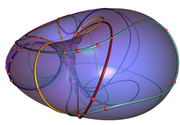max planck institut
informatik# Computational Geometry and Geometric Computing - Winter Term 2009/2010

## Overview:

Geometric computing refers to computation with geometric objects such as points, lines, hyperplanes, disks, curves, surfaces, and solids. These objects live in an ambient space. In this course, ambient space will be mainly two- and three-dimensional Euclidean space. Geometric computing is ubiquitous; the notes for the first lecture give examples.

We discuss important geometric algorithms and their implementation

## Basics and Dates:

• First lecture: October 16, 2009
• No lectures: December 21+25+28, 2009; January 01, 2010
• Last lecture: February 5, 2010
• Tutorial(s): Wednesday, 10-12, Room 023 (E1 4 - MPI for Informatics)
• Tutors: Lecturers

## Information and Rules:

This is a 9-credit-point class.

This course is intended for graduate students and/or senior undergraduate students. It consists of two two-hour lectures and a two-hour problem solving session per week.

The grade is determined by the final exam. The final exam will be oral. We will have exams on February 11th and 12th (in Kurt Mehlhorn's office): NEW: Exact schedule with initials of student's names.

### Exercises:

• Every student has to present the solution to two assignments (typically ~3 per sheet) in the tutorial sessions.
• Every student has to prepare "latex'ed" (sources + pdf) sample solutions to two assignment sheets. Sample solutions are due one week after discussing them in the tutorial. Submitted sample solutions will be checked, and we may ask for improvements.
• The student's "process slip" (hand out in lecture, contact us, if you do not have one) has four empty entries - two for presenting an assigment, two for successful submission of the sample solutions to specified sheets. Admittance to the final exam is granted with four signatures by the lecturers.
• Here is the list of assignements to be solved by students (identified with initials).

## Literature:

Books

• Michael Kerber: Geometric Algorithms for Algebraic Curves and Surfaces. PhD Thesis, Saarland University, 2009 (pdf, ps)
• Mark de Berg, Otfried Cheong, Marc van Kreveld, Mark Overmars: Computational Geometry: Algorithms and Applications
• Jacob E. Goodman, Joseph O'Rourke (Editors): Handbook of Discrete and Computational Geometry
• Jean-Daniel Boissonnat, Monique Teillaud (Editors): Effective Computational Geometry for Curves and Surfaces
• Kurt Mehlhorn, Stefan Näher: LEDA: A Platform for Combinatorial and Geometric Computing
• Matthew H. Austern: Generic Programming and the STL: Using and Extending the C++ Standard Template Library
• C. G. Gibson: Elementary Geometry of Algebraic Curves: An Undergraduate Introduction
• C. Yap: Fundamental Problems in Algorithmic Algebra